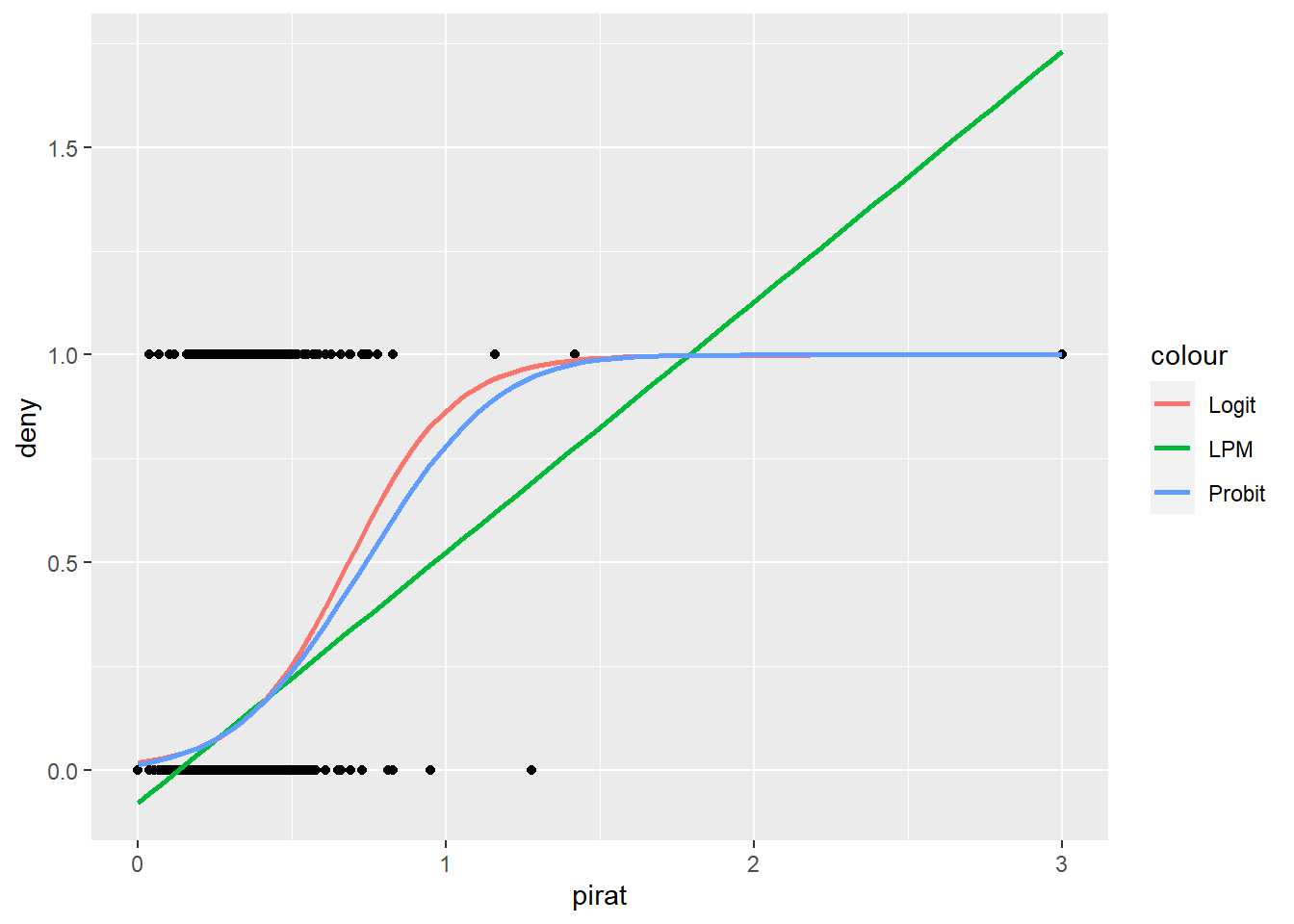# Marginal Effects and Stargazer

This tutorial shows how to put margins output into a stargazer tableImage credit: Unsplash/Lazarescu Alexandra

## The Problem

Though the stargazer package is widely used and an excellent and flexible way of creating tables with different model classes. It does not integrate output from the, equally popular, margins package.

library(tidyverse) # for %>%
library(margins) # for marginal effects
library(AER) # for HMDA data
library(stargazer) # for tables

Let´s use the Home Mortgage Disclosure Act Data from the AER package as an example. Here we try to predict if a mortgage was denied.

# Read Data on Denial of Mortgages
data(HMDA)
Transform Data to Numeric (for LPM)
df <- HMDA %>%
mutate(deny = ifelse(deny == "no", 0, 1))

First let’s explore the relationship between mortgage denial deny and Payments to income ratio pirat.Next, let’s run some models.

# Linear Probability Model
lpm <- lm(deny ~ pirat + hschool + afam,
data = df)
logit <- glm(deny ~ pirat + hschool + afam,
data = df,
family = "binomial")
probit <- glm(deny ~ pirat + hschool + afam,
data = df,
family = binomial(link = "probit"))

We can contrast all of them in a table using the stargazer package.

stargazer(lpm, probit, logit,
type = "html")
 Dependent variable: deny OLS probit logistic (1) (2) (3) pirat 0.549*** 2.743*** 5.365*** (0.060) (0.381) (0.728) hschoolyes -0.097* -0.468** -0.805** (0.051) (0.229) (0.399) afamyes 0.176*** 0.701*** 1.258*** (0.018) (0.084) (0.147) Constant 0.009 -1.800*** -3.333*** (0.056) (0.263) (0.471) Observations 2,380 2,380 2,380 R2 0.077 Adjusted R2 0.076 Log Likelihood -795.139 -793.868 Akaike Inf. Crit. 1,598.277 1,595.737 Residual Std. Error 0.312 (df = 2376) F Statistic 66.481*** (df = 3; 2376) Note: p<0.1; p<0.05; p<0.01

Now let’s get the marginal effects (AME) of the probit model.

summary(margins(probit))
##      factor     AME     SE       z      p   lower  upper
##     afamyes  0.1671 0.0242  6.9135 0.0000  0.1197 0.2145
##  hschoolyes -0.1077 0.0636 -1.6918 0.0907 -0.2324 0.0171
##       pirat  0.5002 0.0688  7.2686 0.0000  0.3653 0.6351

## A (not so elegant) solution

We can impute the AME coefficients to the original model object probit.

probit -> old_model
if(old_model$family$link %in% c("logit", "probit")){
The package that is needed
require(margins)
Some redundancy (just in case you run twice!)
new_model <- old_model
Extract the margins coefficients (named numeric array)
marginal_coefficients <- summary(margins(old_model))$AME Match margins coefficients to model object by (variable) name for(i in names(marginal_coefficients)) { new_model$coefficients[[i]] <- marginal_coefficients[[i]]
}
If the model is neither probit nor logit
} else {print("Invalid Model")}
probit_margins <- new_model

summary(probit_margins)
##
## Call:
## glm(formula = deny ~ pirat + hschool + afam, family = binomial(link = "probit"),
##     data = df)
##
## Deviance Residuals:
##     Min       1Q   Median       3Q      Max
## -2.1145  -0.4772  -0.4253  -0.3518   2.8879
##
## Coefficients:
##             Estimate Std. Error z value Pr(>|z|)
## (Intercept) -1.79983    0.26291  -6.846  7.6e-12 ***
## pirat        0.50021    0.38051   1.315   0.1886
## hschoolyes  -0.10765    0.22942  -0.469   0.6389
## afamyes      0.16709    0.08355   2.000   0.0455 *
## ---
## Signif. codes:  0 '***' 0.001 '**' 0.01 '*' 0.05 '.' 0.1 ' ' 1
##
## (Dispersion parameter for binomial family taken to be 1)
##
##     Null deviance: 1744.2  on 2379  degrees of freedom
## Residual deviance: 1590.3  on 2376  degrees of freedom
## AIC: 1598.3
##
## Number of Fisher Scoring iterations: 5

Unfortunately, our standard errors have not been updated! We can extract them into a separate object.

se_probit <- summary(margins(probit))\$SE
names(se_probit) <- str_remove_all(names(se_probit), "Var_dydx_")
se_probit
##    afamyes hschoolyes      pirat
## 0.02416841 0.06363396 0.06881847
# List of Custom Standard Errors (for stargazer)
custom_se <- list(sqrt(diag(vcov(lpm))),
se_probit)

PseudoR2(probit,"McFaddenAdj")
## McFaddenAdj
##  0.08364626
# Custom list of Adj. R2 for stargazer
"",
))

## The Result

Now we can create a table which compares both models using stargazer!

# Our Comparative Table
stargazer(lpm, probit_margins,
type = "html",
# Our custom r2
se = custom_se)